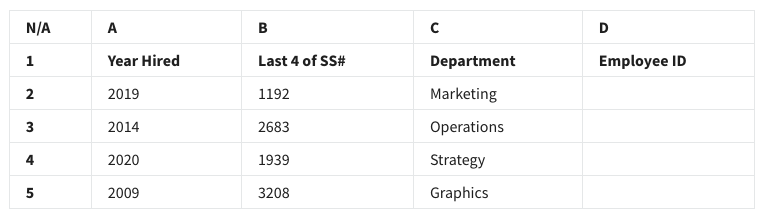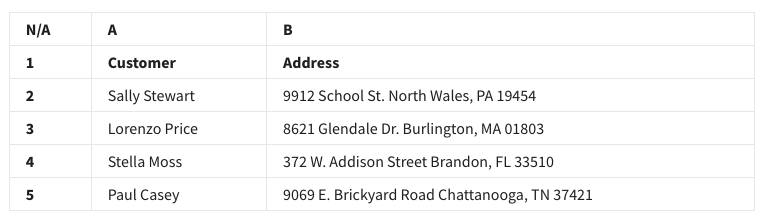## Week 2 – Sparkling-clean data – Shuffle Q/A 1

#### 11. A data analyst is using a function in a spreadsheet. For the function to work correctly, they follow the function’s syntax. What does this entail?

• It is the function’s name and placement.
• It is how the function can be used in a program.
• It is the function’s required information and its proper placement.
• It is the purpose of the function and its use.

• =LEFT(A7,2)
• =LEFT(2,A7)
• =RIGHT(A7,2)
• =RIGHT(2,A7)

• return
• replace
• transform
• delete

#### 14. What describes syntax?

• It is the function’s required information and its proper placement.
• It is how the function can be used in a program.
• It is the purpose of the function and its use.
• It is the function’s name and placement.

#### 15. A data analyst in a human resources department is working with the following selection of a spreadsheet:#### They want to create employee identification numbers (IDs) in column D. The IDs should include the last four digits of the employee’s Social Security Number(SS#) plus the year hired. What function will create the ID 19392020 for the employee in row 4?

• =CONCATENATE(B4+A4)
• =CONCATENATE(B4,A4)
• =CONCATENATE(A4+B4)
• =CONCATENATE(A4!B4)

#### 16. An analyst is cleaning a new dataset. They want to determine how many of the cells in column F have a value of 0. However, they only want rows 7 to 120 to be considered. Which COUNTIF function syntax can be used to answer this question?

• =COUNTIF(F2:F1250, 0)
• =COUNTIF(F7:F120, =0)
• =COUNTIF(F7:F120,”0″)
• =COUNTIF(F7:F120,”=0”)

#### 17. A data analyst needs to combine two datasets. Each dataset comes from a different system, and the systems store data in different ways. What can the data analyst do to ensure the data is compatible prior to analyzing the data?

• Use a data visualization
• Map the data
• Spot check for null values
• Apply a data structure

• Partition
• Delimiter
• Substring
• MID function

## Shuffle Q/A 2

#### 19. A data analyst is using a function in a spreadsheet. When they input the function, they follow a predetermined structure that includes all required information for the function and its proper placement. What aspect of a function does this describe?

• The specified value of the function
• The syntax of the function
• The length of the function
• The number of characters in the function

#### 20. You are working with the following selection of a spreadsheet:• =RIGHT(5,B4)
• =RIGHT(B4,5)
• =LEFT(B4,5)
• =LEFT(5,B4)